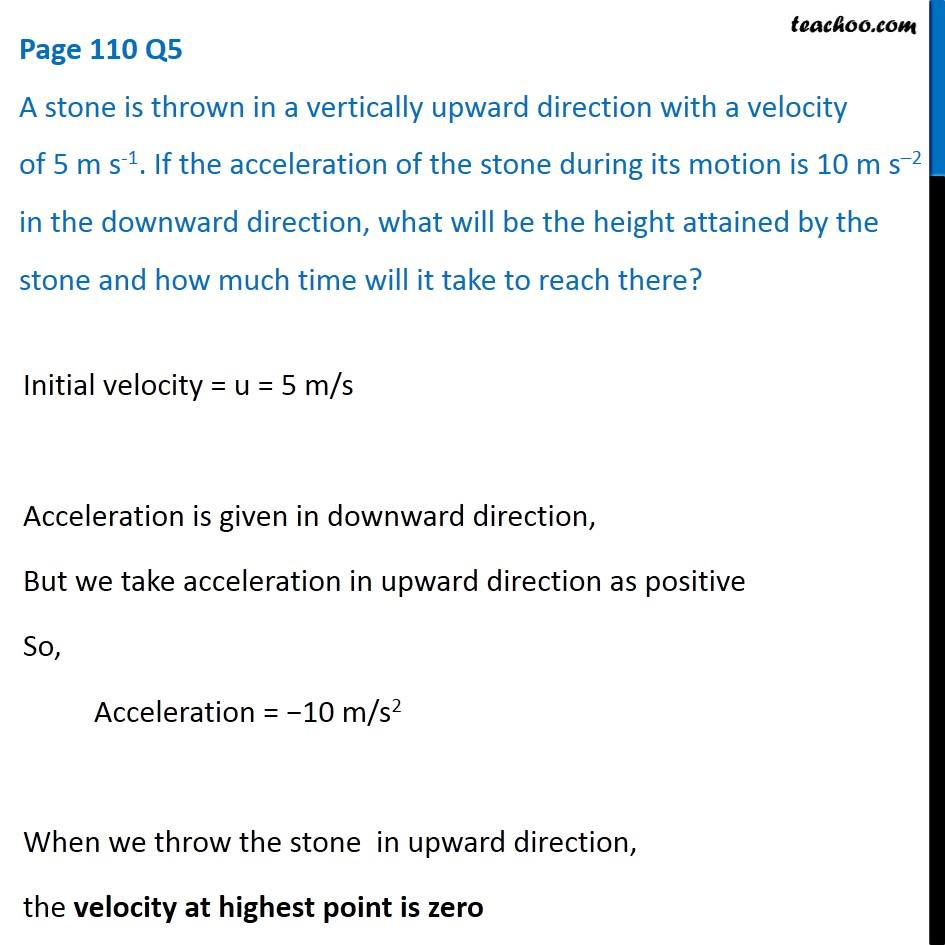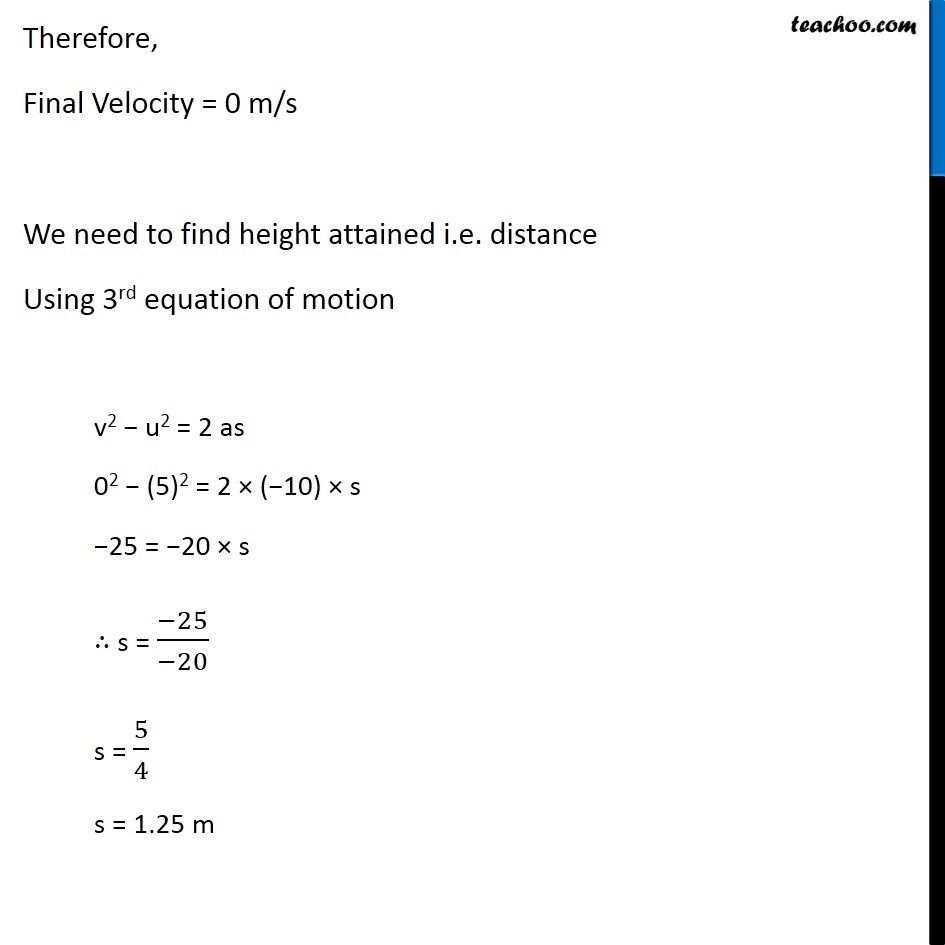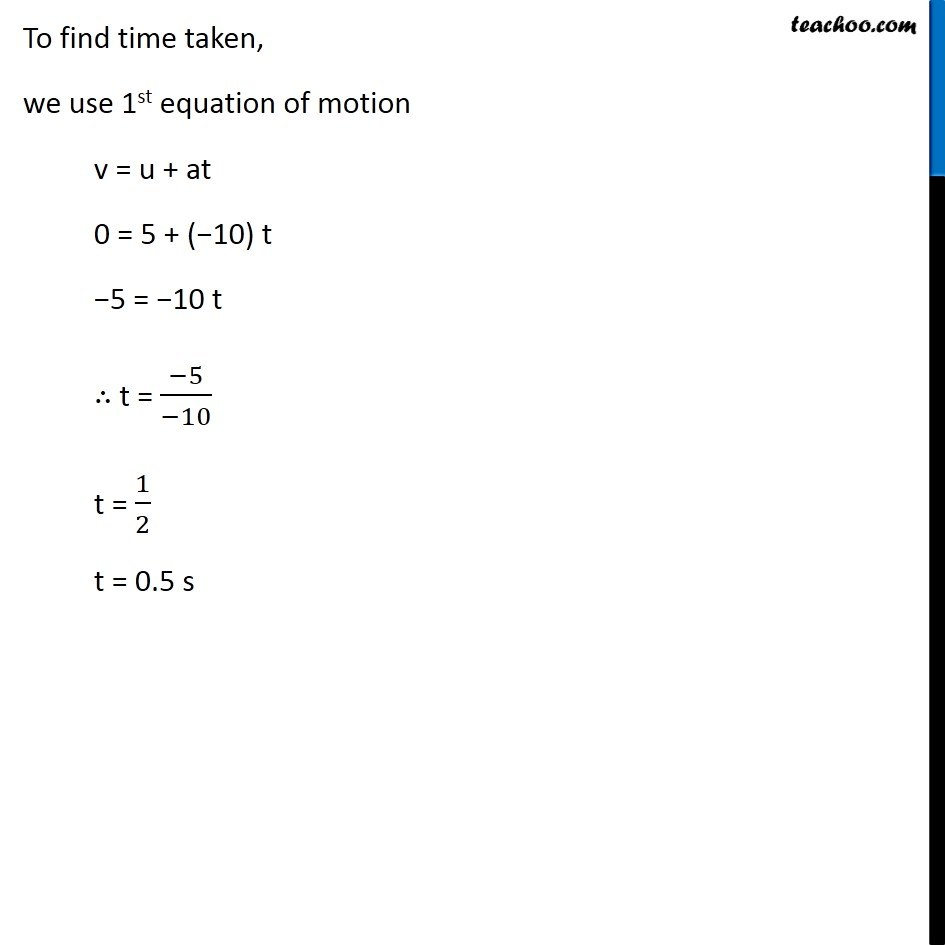Questions from Inside the chapter

Class 9
Chapter 8 Class 9 - MotionLearn in your speed, with individual attention - Teachoo Maths 1-on-1 Class

### Transcript

Page 110 Q5 A stone is thrown in a vertically upward direction with a velocity of 5 m s-1. If the acceleration of the stone during its motion is 10 m s–2 in the downward direction, what will be the height attained by the stone and how much time will it take to reach there? Initial velocity = u = 5 m/s Acceleration is given in downward direction, But we take acceleration in upward direction as positive So, Acceleration = −10 m/s2 When we throw the stone in upward direction, the velocity at highest point is zero Therefore, Final Velocity = 0 m/s We need to find height attained i.e. distance Using 3rd equation of motion v2 − u2 = 2 as 02 − (5)2 = 2 × (−10) × s −25 = −20 × s ∴ s = (−25)/(−20) s = 5/4 s = 1.25 m